## Pricing Table Particle

• Basic
• Standard Compliant Channels
• $50 • Completely synergize resource taxing relationships via premier market • 1 GB of space • Support at$25/hour
• Standard Compliant Channels
• $100 • Completely synergize resource taxing relationships via premier market • 10 GB of space • Support at$15/hour
• Platinum
• Standard Compliant Channels
• $250 • Completely synergize resource taxing relationships via premier market • 30 GB of space • Support at$5/hour

### Search Our Site

Measurement 2: Surface Area and Volume
Mathematics
Topic Six

# Surface Area

The surface area of a figure is the total area of all the sides of the figure.

### Triangular Prism (Right Angle Triangle)To find the surface area of the prism above, follow the steps below:

Divide the figure into smaller shapes, find the area of each smaller shape and then sum the areas of each smaller shape.  A right-angle triangle prisim can be divided into five smaller shapes which are two right angle triangles and three rectangles.

Example

Find the surface area of the prism below.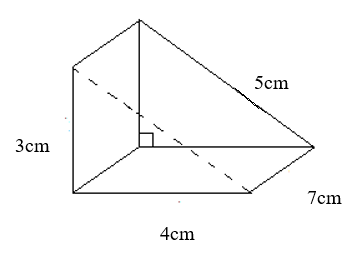Area of right angle triangle = 1/ 2 base  x  height = 1/ 2 x 4 x 3 = 6

As there are 2 of these right angle triangles, we have 6 x 2 = 12

Area of rectangle 1:  4 x 7=  28

Area of rectangle 2:  5 x 7=  35

Area of rectangle 3:  3 x 7= 21

Surface area = 12 + 28  + 35 + 21 = 96cm2

### Pyramid

A pyramid is a solid formed by connecting a polygonal base and a point, called the apex.

The surface area of a pyramid = Area of the base + 1/ 2 Perimeter of Base x Length of Side

Example

Find the surface area of the following pyramid

Surface area of pyramid = Area of base + 1/ 2 perimeter of base x lengthArea of 6 x 6 = 36cm2

Perimeter = 1/ 2  4(6) = 12cm

Surface area = 36 + 12 x 12 =  36 + 144 = 180 cm2

### Cylinder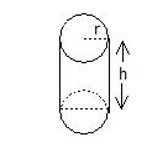A cylinder can be divided into three parts: two circles and a curved surface area.

So the surface area = 2πr2 + 2πrh

The first term is the area of the two circles and the second term is the perimeter of the cylinder.

Example

Find the surface area of a cylinder with a radius of 3 cm, and a height of 2 cm.

### SolutionSA = 2 × π × r2  +   2 × π × r × hSA = 2 × 3.14 × 32  +   2 × 3.14 × 3 × 2SA = 6.28 × 9  +   6.28 × 3 x 2SA = 56.52 + 37.68Surface area = 94.2 cm2Cube

A cube has 6 sides or faces of the same surface area. Therefore, if the area of a square is side times side, then the surface area is S times S times 6.

### Cuboid

cuboid is an object with six flat faces and all angles are right angles with all its faces being rectangles.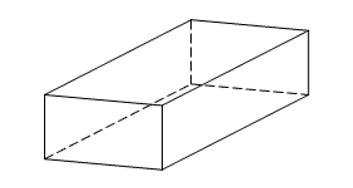The surface area of a cuboid, A =  2Lw + 2 Lh + 2wh

where,

L is the length of the cuboid

w is the width and

h is the height of the cuboid.

Example

Find the surface area of a cuboid with length of 5cm, width 6cm and height 7cm.

Solution

A =  2Lw + 2 Lh + 2wh

= 2 x 5 x 6 + 2 x 5 x 7 + 2 x 6 x 7

= 60 + 70 + 84

= 214cm2

### Sphere

A sphere is a three-dimensional object such as a ball or the globe with every point on the surface halfway from the center.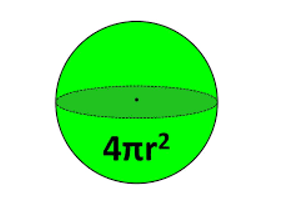The surface area of a sphere, A = 4πr2

where, r is the radius of the sphere.

Example

Find the surface area of a sphere with radius of 9cm.

Solution

Surface Area of a sphere, A = 4 πr2

4 x 3.14 x 9 x  9 = 1018 cm2

# Volume

Volume is the amount of 3-dimensional space an object occupies.

Cube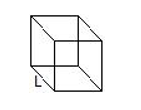The volume of a cube , V = L3

where, L is the length of a side of the cube.

Example

The volume of a cube with side of 4cm = 4 x 4 x 4 = 64cm3

### CuboidThe volume of a cuboid, V = Lwh

where, L is the length of the cuboid,

w is the width and h is the height of the cuboid.

### Sphere

The volume of a sphere, V = 4/3 πr3

where, r is the radius of the sphere.

Example

The volume of a sphere with radius of 7cm = 4/ 3 x 22/ 7 x 7 x 7 x 7 = 4/ 3 (22/ 7) 7x7x7 = 1436.9cm3

### Triangular Prism (Right Angle Triangle)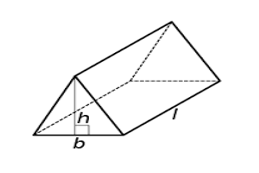The volume of a triangular prism, V =  Area of one of the triangles times length

= 1/ 2 base x height x length of the triangular prism

Example

Find the volume of a triangular prism with base of 6cm, length of 8cm and height of 7cm.

Solution

Volume = 1/  2 (6) x 7 x 8

= 3 x 7 x 8 = 168cm3

### Pyramid

The volume of a pyramid = 1/ 3 Area x height.

Example

Find the volume of a pyramid with length of 9cm and height of 14cm.

Solution

V = 1/ 3 (9 x 9) x 14 =

= 27 x 14 = 378cm3

### Cylinder

The volume of a cylinder, V =  πr2h

where, πr2 is the area of the circular cross-section and h is the height of the cylinder.

Example

Find the volume of a cylinder with radius of 7 cm and height of 14cm.

Solution

Volume = 22/ 7 x 7 x 7 x 14

= 154 x 14 = 2155cm3

# Distance, Speed and Time

There are mathematical problems that require students to be able to calculate distance, time and speed.

Distance = Speed x Time

Time = Distance/Speed

Speed = Distance/Time

### Distance

Example

Joe drove from his home to the mall at an average speed of 50 kilometres an hour and took 4 hours to do so.  Calculate the distance from his home to the mall.

Solution

Distance = Speed x Time = 50 x 4 = 200 kilometres.

### Speed

Example

Sherry walked to the park located 80 kilometers in 5 hours.  Calculate her speed per hour.

Speed = Distance/Time = 80/5 = 16 kilometers per hour.

### Time

A lady travelled a distance of 45 kilometers at a speed of 5 kilometers per hour.  Calculate the time she took.

Speed = Distance/ Speed = 45/5 = 9 hours